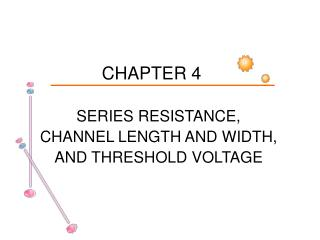Download PresentationCHAPTER 4

# CHAPTER 4 - PowerPoint PPT PresentationDownload Presentation## CHAPTER 4

- - - - - - - - - - - - - - - - - - - - - - - - - - - E N D - - - - - - - - - - - - - - - - - - - - - - - - - - -
##### Presentation Transcript

1. CHAPTER 4 SERIES RESISTANCE, CHANNEL LENGTH AND WIDTH, AND THRESHOLD VOLTAGE

2. 4.1 INTRODUCTION

3. Semiconductor devices is degraded by series resistance. • Series resistance depends on semiconductor resistivity, contact resistance, and the geometrical factors.

4. 4.2 PN JUNCTION DIODES

5. Current-Voltage

6. Equivalent circuit of a diode.

7. Log(Ι) versus V for a diode with series resistance. The upper dashed line is for rs=0

8. Open-Circuit Voltage Decay Open-circuit voltage decay of a pn junction showing the voltage discontinuity at t=0

9. Capacitance-Voltage 1. f low, such that 2πfrsC<<1, to obtain C 2. f high, such that 2πfrsC>>1,calculate rs from the data of Cm vs. f. 3. This method is used when DC method is unavailable, such as in MOS capacitor.

10. 4.3 SCHOTTKY BARRIER DIODES

11. Series Resistance The method of extracting rs for pn diode can also be used for Schottky diode.

12. Norde function F is defined as: It can also be written as: For low voltage Irs<<V, dF/dV≒1/2-1=-1/2 For high voltage Irs ≒V, dF/dV≒-1/2+1=1/2 Therefore, there exists a Fmin. From Fmin. to determine Vmin. and then find out Imin..

13. Another method is define H as: Plot H vs. I, the slope is rs and the intercept is nψB.

14. A modified Norde function is defined as: Plot F1 vs. V for different Temp., each curve has a F1min , which has a corresponding Vmin and Imin. Plot the left side of the above equation vs. q/kT, then n and A* can be extracted from the slope and the intercept, respectively.

15. 4.4 SOLAR CELLS

16. SOLAR CELLS Equivalent circuit of a solar cell.

17. Multiple Light Intensities Current-voltage characteristic of a solar cell.

18. Series resistance determination of a solar cell.

19. For Idk=Iph=Isc

20. Constant Light Intensity This method (area method) is suitable for concentrator cells with high Iph, it overestimates rs at 1-sun. This method (area method) is suitable for concentrator cells with high Iph, it overestimates rs at 1-sun.

21. Constant Light Intensity For high intensity flash illumination method, neglecting rsh, I≒Voc/(RL+rs). By varying the load resistance RL at constant light intensity, we have RL should be on the order of rs.

22. Shunt Resistance Rewrite the above equation as

23. For rs<<rsh and Iscrs<<nkT/q, rs≒0.1Ω, Isc≦3mA it becomes At very low light intensities, the second term is neglected, then

24. 4.5 BIPOLAR JUNCTION TRANSISTORS

25. BIPOLAR JUNCTION TRANSISTORS An npn bipolar junction transistor and its parasitic resistances. The base resistance is composed of intrinsic and extrinsic resistance.

26. Gummel plots showing the effects of emitter-base space -charge region recombination (n≈2), quasineutral region recombination (n≈1), and series resistance.

27. Emitter Resistance RE≒1Ω for discrete BJT and around 5~100Ω for IC transistors. Emitter resistance measurement setup and IB -VCE plot.

28. Emitter Resistance Another method is to supply current from B1 only, and no current flow through B2. Then VBE2=VBEeff+IERE. RE=(VBE2-VBEeff)/IE Where

29. Collector Resistance Common emitter output characteristics. The two lines show the limiting values of RC .

30. Base Resistance Plot ΔVBE/IB vs β has a slope of RE and an intercept on the ΔVBE/IB axis of RB+RE. But it is difficult to change β of a transistor without changing other parameters.

31. Base Resistance Rewrite the equation of IB in the following form Measured device characteristics for a self-aligned, high-speed digital BJT. The βmust be varied in this measurements, and RBi/β must be a constant.

32. Base Resistance The intrinsic base resistance for rectangular emitter with 1 contact is: RBi=RshiW/3L. The intrinsic base resistance for rectangular emitter with 2 contact is: RBi=RshiW/12L. Square emitter with contact on all sides: RBi=Rshi/32. (W=L) Circular emitter with contactall around: RBi=Rshi/8π.

33. Equivalent emitter-base portion of the “two-base contact” BJT.

34. Measured base resistance versus emitter window width as a function of base-emitter voltage. d is the deviation between emitter window and the effective base width. Rshi is a function of VBE due to base conductivity modulation.

35. Frequency Measurements on RB The input impedance circle method measures the Zin as a function of frequency. The real axis intersections give:

36. 4.6 MOSFETs

37. Series Resistance and Channel Length— I-V ξ=0.37, 0.58, 0.75, 0.9; xch: channel thickness • A MOSFET with source and drain resistances, (b) device cross • section showing the actual gate length L and Leff =L-∆L with • ∆L=2δL. The substrate resistance is not shown.

38. Method 1

39. Rm versus L as a function of gate voltage.

40. Short channel devices have a channel length dependent threshold voltage. • VG↗, Leff ↗, RSD↘. • Keep VG at a fixed value, change VT by changing VSB.